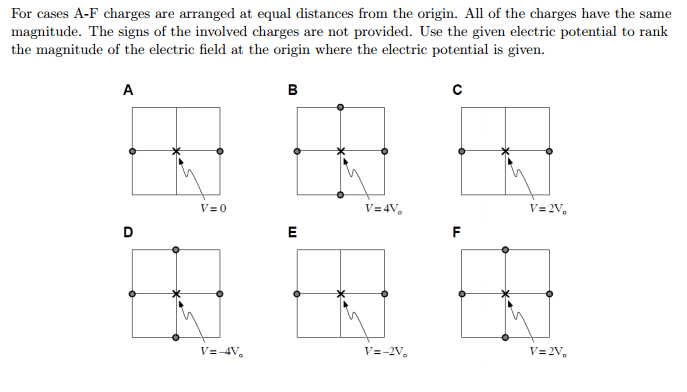# Electric field and electric potential

## Homework Statement[/B]

dV = -EdX[/B]

## The Attempt at a Solution

Well I was trying to think about the relationship between E and V. So I believe the two V and E are co dependent. So in a situation where the distance and the potential are not changing then wouldn't V just be equal to -E? If that is correct then: D>E>A>F=C>B is the answer.[/B]

gneill
Mentor
The difference is that while potentials add like numbers, fields add like vectors. That's because potential is a scalar quantity while fields are vector quantities. Two fields of equal magnitude can cancel each other out simply by being pointed in opposite directions.

The difference is that while potentials add like numbers, fields add like vectors. That's because potential is a scalar quantity while fields are vector quantities. Two fields of equal magnitude can cancel each other out simply by being pointed in opposite directions.

gneill
Mentor
Nope. You'll have to go through the diagram and assign some signs to the charges that work for the potential given, then add up the field contributions (as vectors).

Nope. You'll have to go through the diagram and assign some signs to the charges that work for the potential given, then add up the field contributions (as vectors).

I am a little confused like for case B if I say all are + and I know the value of the charges are say q for each then E is going to be zero for the point x right because all the vectors cancel. However, using that logic on case F confuses me. The two cases seem to be similar but the charges would have to be different than say q like in case B but the directions say all the charges are the same.

Something else that still confuses me is assigning signs to the charges. Like I know how to figure out the over-all pe of a system of particles but not of a system at a designated point.

Last edited:
gneill
Mentor
I am a little confused like for case B if I say all are + and I know the value of the charges are say q for each then E is going to be zero for the point x right because all the vectors cancel. However, using that logic on case F confuses me. The two cases seem to be similar but the charges would have to be different than say q like in case B but the directions say all the charges are the same.
The charges all have the same magnitude but may have different signs. Case F can be solved with the correct application of signs to the charges. You know that the net potential at the origin is positive, so there must be an excess of positive charges in the scenario. So start with them all positive and then change one... If nothing else you could try trial and error :)
Something else that still confuses me is assigning signs to the charges. Like I know how to figure out the over-all pe of a system of particles but not of a system at a designated point.

The potential in this case is the electric potential (Volts). Any point in space has an electric potential due to the net effect of all the charges that exist. Fortunately most of them cancel out!

Okay so here is what I am thinking now:

A=F>B=C=D=E

is that correct?

gneill
Mentor
I have to say that I'm not keen on just validating what could be guesswork without seeing the details of the work. But I get the feeling that you've put in the effort and understood what you're doing. So in this instance I will confirm that your answer is good :)

I have to say that I'm not keen on just validating what could be guesswork without seeing the details of the work. But I get the feeling that you've put in the effort and understood what you're doing. So in this instance I will confirm that your answer is good :)